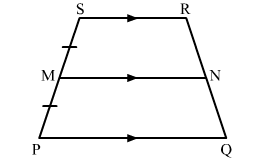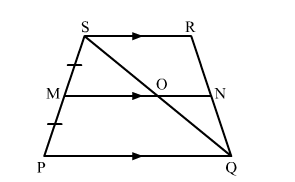# In the adjoining figure, PQRS is a trapezium in which PQ || SR and M is the midpoint of PS. A line segment MN || PQ meets QR at N.

Question:

In the adjoining figure, PQRS is a trapezium in which PQ || SR and is the midpoint of PS. A line segment MN || PQ meets QR at N. Show that N is the midpoint of QR.Solution:Given: In trapezium PQRS, PQ || SR, is the midpoint of PS and MN || PQ.

To prove: N is the midpoint of QR.

Construction: Join QS.

Proof:

In SPQ,

Since, M is the mid-point of SP and MO || PQ.

Therefore, O is the mid-point of SQ.      (By Mid-point theorem)

Similarly, in SRQ,

Since, O is the mid-point of SQ and ON || SR           (SR || PQ and MN || PQ)

Therefore, N is the mid-point of QR.      (By Mid-point theorem)